Neverending Pun Machine

# npm

## ode-midpoint

1.0.6 • Public • Published

# ode-midpointIntegrate a system of ODEs using the Second Order Runge-Kutta (Midpoint) method

## Introduction

This module integrates a system of ordinary differential equations of the form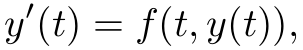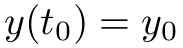where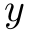is a vector of length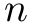. Given time step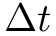, the midpoint method integrates the ODE with update## API

### `require('ode-midpoint')( y0, deriv, t0, dt )`

Arguments:

• `y0`: an array or typed array containing initial conditions. This vector is updated in-place with each integrator step.
• `deriv`: a function that calculates the derivative. Format is `function( dydt, y, t )`. Inputs are current state `y` and current time `t`, output is calcualted derivative `dydt`.
• `t0`: initial time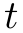.
• `dt`: time step.

Returns: Initialized integrator object.

Properties:

• `n`: dimension of `y0`.
• `y`: current state. Initialized as a shallow copy of input `y0`.
• `deriv`: function that calcualtes derivative. Initialized from input. May be changed.
• `t`: current time, incremented by `dt` with each time step.
• `dt`: time step. Initialized from input `dt`. May be changed.

Methods:

• `.step()`: takes a single step of the midpoint integrator and stores the result in-place in the `y` property.
• `.steps( n )`: takes `n` steps of the midpoint integrator, storing the result in-place in the `y` property.

## Credits

(c) 2015 Ricky Reusser. MIT License

## Keywords

### Install

`npm i ode-midpoint`

### Repository

github.com/scijs/ode-midpoint